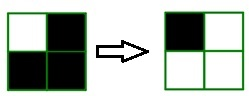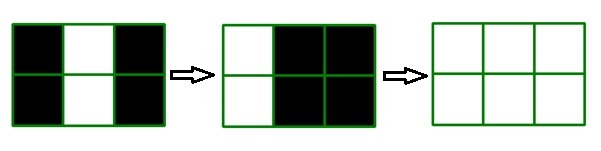Mosaics and holes
Tag(s):

## Algorithms, Easy, Greedy algorithm, Greedy algorithm, Greedy algorithm

Problem
Editorial
Analytics

John wants to cover his yard floor with mosaics. The yard floor is a $n \times m$ matrix and each cell is either a mosaic or a hole. John has invented a flipper machine. This machine has a size of $k$ and can select a $k \times k$ square of the yard and flip the cells in it. By this action, every hole in the square becomes a mosaic and every mosaic in the square becomes a hole. It is illustrated by the following example:The $2 \times 2$ square on the left has been flipped to the right one by the flipper with the size $2$ and blacks are holes and whites are mosaics.

Help John to cover the floor of the yard completely by mosaics by using this machine. In each step, John selects a $k \times k$ square of the yard floor and sets the machine on it. He wants to compute the minimum steps needed to repair the yard floor.

Input Format

• First line: Three integers $n, m, k$ ($1 \leq n, m \leq 1000 , 1 \leq k \leq min(n,m)$), where $n$ is the number of matrix rows, $m$ as the number of matrix columns, and $k$ as the size of the flipper machine.
• Next n lines: Each line will contain m integer 0 or 1 with space in between the consecutive one.
• This $n$ lines are the data of the yard floor matrix, 1 indicates that the cell is mosaic and 0 indicates that the cell is a hole.

Output format

Print an integer denoting the minimum number of steps needed to repair the yard floor or -1 if it is impossible to repair the yard floor with this machine.

SAMPLE INPUT
2 3 2
0 1 0
0 1 0

SAMPLE OUTPUT
2

Explanation

we can repair the yard by 2 steps as shown below.you can see more sample tests here!

 Sample Input Sample output 3 3 2 0 0 0 0 1 0 0 0 0 -1 2 2 1 0 0 0 0 4

Time Limit: 1.0 sec(s) for each input file.
Memory Limit: 256 MB
Source Limit: 1024 KB
Marking Scheme: Marks are awarded when all the testcases pass.
Allowed Languages: Bash, C, C++, C++14, Clojure, C#, D, Erlang, F#, Go, Groovy, Haskell, Java, Java 8, JavaScript(Rhino), JavaScript(Node.js), Julia, Kotlin, Lisp, Lisp (SBCL), Lua, Objective-C, OCaml, Octave, Pascal, Perl, PHP, Python, Python 3, R(RScript), Racket, Ruby, Rust, Scala, Swift, Swift-4.1, TypeScript, Visual Basic

## CODE EDITOR

Initializing Code Editor...

## This Problem was Asked inChallenge Name

June Easy' 19

OTHER PROBLEMS OF THIS CHALLENGE
• Basic Programming > Input/Output
• Algorithms > Sorting
• Algorithms > Graphs
• Math > Basic Geometry
• Algorithms > Graphs